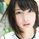5118 views
5118
3 lines RCI
Original author is Jadbrother and modified for 3 lines, 9,35,52, by gero on Jun.29 2016.
```//
// @author Jadbrother modified by gero
//
//@version=2
study(title = "RCI3lines", shorttitle = "RCI3lines")

itvs = input(9, "short interval")
itvm = input(36, "middle interval")
itvl = input(52, "long interval")
src = input(close, "source")
upperband=input(title="High line[%]",defval=80,type=integer)
lowerband=input(title="Low line[%]",defval=-80,type=integer)

ord(seq, idx, itv) =>
p = seq[idx]
o = 1
for i = 0 to itv - 1
if p < seq[i]
o := o + 1
o

d(itv) =>
sum = 0.0
for i = 0 to itv - 1
sum := sum + pow((i + 1) - ord(src, i, itv), 2)
sum

rci(itv) => (1.0 - 6.0 * d(itv) / (itv * (itv * itv - 1.0))) * 100.0

hline(upperband,color=gray,linestyle=dashed)
hline(lowerband,color=gray,linestyle=dashed)
plot(rci(itvs), title = "RCI short", color = red)
plot(rci(itvm), title = "RCI middle", color = blue)
plot(rci(itvl), title = "RCI long", color = green)
```Hi, gero. My name is Jadbrother. I found that your script was used by a lot of people, and seemed to modify my script ( ).

My original script had a bug, so I published fixed version ( ) before, but unfortuately you looked using my original buggy script.

So I recommend that you fix your script. Please look at my fixed ord() function carefully.

Regards,